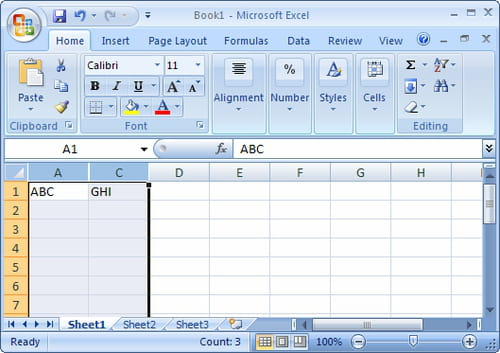# MOREFUNC (Macro add-in for EXCEL)

3 vote - 5.0 /5
Developer:
Version:
5.06
Windows XP Windows Vista Windows 2000 - English
10/10

The functions are compatible only with Excel 95-2007.

Posts
1150
Registration date
Wednesday November 4, 2020
Status
Last seen
September 20, 2021

MOREFUNC is an add-in for Excel offering 67 new worksheet functions for Excel.

These functions are compatible with Excel 95-2007. They are only portable on Windows OS.

This add-in is written in C++ and is stored in an XLL file, i.e a DLL capable of interfacing with Excel. The speed of compiled code provides a swift calculation which is quite similar to the integrated Excel functions.## Key features

### Date and time

• DATEDIFF: returns the elapsed time between two dates in years, months and days
• DIMPAQUE: returns the date of Easter Sunday for a given year
• WEEKNUM: Calculates the week number of a date (according to ISO standard)

### Information

• EVA: Evaluates a given expression as text
• INDIRECT.EXT: returns the contents of a cell or a range contained in a closed workbook
• MRFVERSION: returns MOREFUNC version number
• WORKBOOKNAME: returns the name of the current workbook
• SHEETNAME: returns the name of a sheet in the current workbook
• PAGENUM: returns the page number of a cell
• TEXTEFORMULA: returns the formula of a cell
• READ.XLM.CELL: returns information about formatting, location or contents of a cell

### Mathematics

• RANDOM: returns a set of integers from 0 or 1 randomly arranged and without repetition
• MAT.ROUND: returns a rounded balanced picture
• CHBASE: converts a value of a digital database to another
• DETERMAT.EXT: returns the determinant of a matrix of big size
• HEX.AND: performs a AND logical function on hexadecimal numbers
• HEX.FORMAT: formats a hexadecimal number to the number of specified bits
• HEX.NEG: reverses the sign of a hexadecimal number
• HEX.NOT: reverse the bits of a hexadecimal number (NOT logical)
• HEX.OR: performs a logical OR of hexadecimal numbers
• HEX.SUM: returns the sum of hexadecimal numbers
• INVERSEMAT.EXT: inverses a matrix of big size
• AVERAGEMARK.GEO.EXT: geometric average mark supporting calculation of capacity
• MOYPOND: returns a weighted average
• NP.ISPRIME: test if a number is prime (probabilistic method)
• NP.NEXT: returns the smallest prime number greater than or equal to the indicated number
• PRODUITMAT.EXT: performs matrix multiplication between large tables

### Statistics

• ECARTYPE.GROUP: evaluates the standard deviation of a population grouped in classes
• ECARTYPEP.GROUP: calculates the standard deviation of a population grouped in classes
• MMAX: returns the N largest values in a table
• MMIN: returns the N smallest values in a table
• NB.DIFF: returns the number of distinct values in a range
• NB.SI.3D: conditional enumeration on a 3D range
• VAR.GROUP: estimates population variance group classes
• VARP.GROUP: calculates population variance group classes

### Text

• ANSI128: removes accents from a string
• MCONCAT: concatenates all values in a range or table
• NB.MOTS: returns the number of words in a text
• NBTEXTE: transcribes a number in words (13 supported languages)
• REGEX.COMP: tests the presence of a regular expression in a text
• REGEX.NBCAR: returns the number of characters in a regular expression found in a text
• REGEX.NUMBER: counts the number of occurrences of a regular expression in a text
• REGEX.STXT: extracts a sub- string using regular expressions
• REGEX.SUBSTITUTE: replaces characters in a text using a regular expression
• REGEX.FIND: returns the position of a regular expression in a text
• STEXT: returns a word or a group of words located at a certain position in a text
• TEXTEINVERSE: reverses the order of characters in a text

### Automatic sorting

• TRIH: sorts the columns in a range or table (up to 14 sort keys)
• TRIH.IDX: returns the index of a horizontal tri
• IVRT: sorts the rows of a table or a range (up to 14 sort keys)
• TRIV.IDX: returns the index of a vertical sorting
• UNIQUE.VALUE: returns the unique values of a data set

### Other functions

• SHIFTWORKSHEET: returns an offset track or multiple sheets
• DERCELL: returns the last non-empty cell or range of worksheet
• DVAR: save value in a temporary variable
• LVAR: recover the value of a temporary variable
• REMINDER: returns the last contents of the calling cell
• REGION: returns the area of a cell (similar to the CurrentRegion method in VBA)
• SUITE: generates an increasing sequence of integers
• TAB.FILTRE: returns only the visible cells in a range
• TAB.JOIN: assemble scattered elements in an array
• TAB3D: assembles the values of a 3D reference in a single table
• MERGE.SHIFT: performs a shift on a union of disjoint ranges